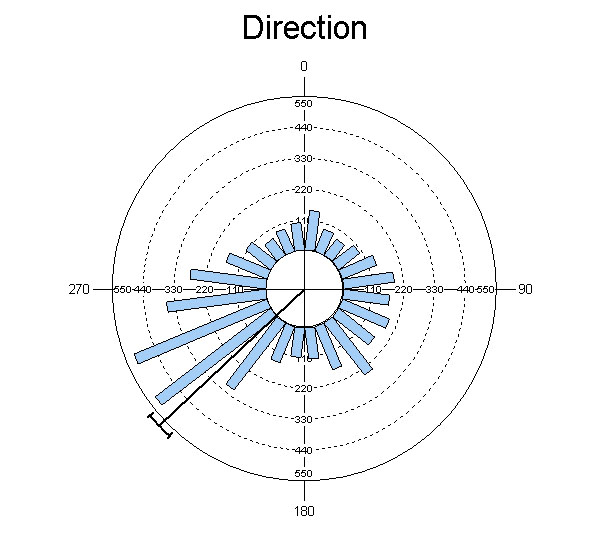# Oriana Analyses

• Basic statistics
• circular mean,
• length of mean vector (r),
• median angle
• circular variance, standard deviation and standard error
• 95% and 99% confidence interval for the mean vector.
• mean weighted by linear variable, plus basic statistics for the linear variable itself
• means and mean vector lengths can be saved to a file for further second order analysis.
• One-sample tests
• Rayleigh’s test of uniformity
• Hotelling’s test for weighted data
• Moore’s modified Rayleigh test for weighted data
• Rao’s spacing test
• Chi-Squared
• V-Test
• Watson’s U²
• Kuiper’s.
The last two can be used for tests against both the uniform and von Mises distribution.
• Two-sample tests
• Watson-Wheeler F-test
• Chi-squared test
• Mardia-Watson-Wheeler test
• Watson’s U² test.
• Hotelling’s paired multisample test
• Moore’s paired multisample test (nonparametric)
• Multisample tests – The first three two-sample tests above can also be performed as multi-sample tests.
• Circular-circular and circular-linear correlations.
• Second order statistical analysis:
• Grand mean angle, length and confidence limits
• Hotelling’s one sample second order test
• Moore’s modified Rayleigh test for second order analyses
• Hotelling’s two sample second order test
• Mardia’s two sample second order test (nonparametric)
• Hotelling’s paired sample second order test
• Moore’s paired sample second order test (nonparametric)
• All analyses can be performed on subgroups of data as well as the whole data set.